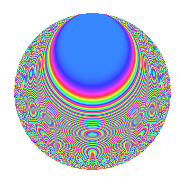# Properties

 Label 287.2.nLevel 287 Weight 2 Character orbit n Rep. character $$\chi_{287}(64,\cdot)$$ Character field $$\Q(\zeta_{10})$$ Dimension 88 Newforms 1 Sturm bound 56 Trace bound 0

# Related objects

## Defining parameters

 Level: $$N$$ = $$287 = 7 \cdot 41$$ Weight: $$k$$ = $$2$$ Character orbit: $$[\chi]$$ = 287.n (of order $$10$$ and degree $$4$$) Character conductor: $$\operatorname{cond}(\chi)$$ = $$41$$ Character field: $$\Q(\zeta_{10})$$ Newforms: $$1$$ Sturm bound: $$56$$ Trace bound: $$0$$

## Dimensions

The following table gives the dimensions of various subspaces of $$M_{2}(287, [\chi])$$.

Total New Old
Modular forms 120 88 32
Cusp forms 104 88 16
Eisenstein series 16 0 16

## Trace form

 $$88q - 24q^{4} + 8q^{5} + 10q^{6} - 18q^{8} - 116q^{9} + O(q^{10})$$ $$88q - 24q^{4} + 8q^{5} + 10q^{6} - 18q^{8} - 116q^{9} + 36q^{10} - 10q^{11} + 20q^{15} - 12q^{16} - 10q^{17} + 20q^{18} + 30q^{19} - 30q^{20} + 4q^{21} - 20q^{22} - 12q^{23} + 60q^{24} - 50q^{25} - 30q^{26} + 2q^{31} + 24q^{32} - 46q^{33} + 50q^{34} + 86q^{36} - 48q^{37} + 16q^{39} - 60q^{40} - 24q^{41} - 4q^{42} + 22q^{43} - 16q^{45} + 20q^{46} + 20q^{48} + 22q^{49} - 16q^{50} + 8q^{51} + 70q^{52} - 30q^{54} + 8q^{57} - 90q^{58} - 4q^{59} - 50q^{60} - 64q^{61} - 44q^{62} + 14q^{64} + 80q^{65} - 26q^{66} + 10q^{67} + 40q^{71} + 18q^{72} + 124q^{73} + 80q^{74} + 70q^{75} - 190q^{76} + 8q^{77} + 74q^{78} + 26q^{80} + 144q^{81} - 58q^{82} - 60q^{83} + 26q^{84} + 10q^{86} + 8q^{87} + 160q^{88} - 164q^{90} - 40q^{91} - 156q^{92} - 20q^{93} + 10q^{94} + 80q^{95} - 90q^{97} - 40q^{99} + O(q^{100})$$

## Decomposition of $$S_{2}^{\mathrm{new}}(287, [\chi])$$ into irreducible Hecke orbits

Label Dim. $$A$$ Field CM Traces $q$-expansion
$$a_2$$ $$a_3$$ $$a_5$$ $$a_7$$
287.2.n.a $$88$$ $$2.292$$ None $$0$$ $$0$$ $$8$$ $$0$$

## Decomposition of $$S_{2}^{\mathrm{old}}(287, [\chi])$$ into lower level spaces

$$S_{2}^{\mathrm{old}}(287, [\chi]) \cong$$ $$S_{2}^{\mathrm{new}}(41, [\chi])$$$$^{\oplus 2}$$